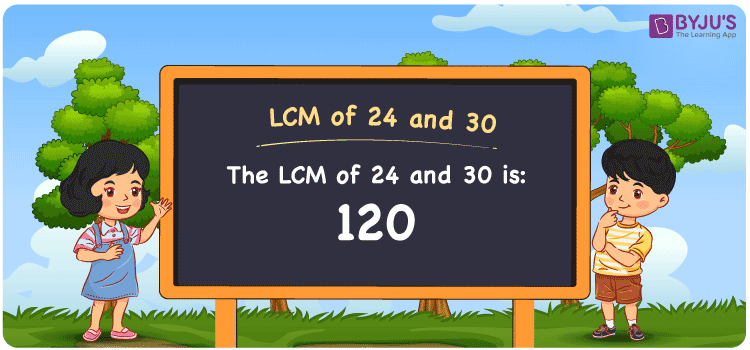Checkout JEE MAINS 2022 Question Paper Analysis : Checkout JEE MAINS 2022 Question Paper Analysis :

# LCM of 24 and 30

LCM of 24 and 30 is 120. The concept of LCM is used to make the simplification process easier while solving problems related to the addition and subtraction of fractions. Hence, it is essential to have core knowledge of the Lowest Common Multiple to solve arithmetic problems with ease. Students are recommended to refer to the article Prime Factorisation and Division Method for LCM and HCF to find the LCM and HCF of given numbers in an efficient manner. Let us learn the simple method to find the least common multiple of 24 and 30 in this article.

## What is LCM of 24 and 30?

The answer to this question is 120.## How to Find LCM of 24 and 30?

The following methods are used to find the LCM of 24 and 30

• Prime Factorisation
• Division method
• Listing the multiples

### LCM of 24 and 30 Using Prime Factorisation Method

By prime factorisation method, we can write 24 and 30 as the product of prime numbers, such that;

24 = 2 × 2 × 2 × 3

30 = 2 × 3 × 5

LCM (24, 30) = 2 × 2 × 2 × 3 × 5 = 120

### LCM of 24 and 30 Using Division Method

Here, we divide the numbers 24 and 30 by their prime factors to determine their LCM. The product of these divisors represents the least common multiple of 24 and 30.

 2 24 30 2 12 15 2 6 15 3 3 15 5 1 5 x 1 1

No more further division can be done.

Hence, LCM (24, 30) = 2 × 2 × 2 × 3 × 5 = 120

### LCM of 24 and 30 Using Listing the Multiples

To calculate the least common multiple of 24 and 30 using listing multiples, we list out the multiples of 24 and 30 as shown below.

 Multiples of 24 Multiples of 30 24 30 48 60 72 90 96 120 120 150 144 180

LCM (24, 30) = 120

## Related Articles

Least Common Multiple (LCM)

LCM of Two Numbers

HCF and LCM

LCM calculator

LCM Formula

LCM with Examples

## Video Lesson on Applications of LCM## Solved Examples

Q. 1: Find the LCM of 24 and 30 using prime factorisation.

Solution: The LCM of 24 and 30 using prime factorisation can be determined as follows

24 = 2 × 2 × 2 × 3

30 = 2 × 3 × 5

LCM (24, 30) = 2 × 2 × 2 × 3 × 5 = 120

Hence the least common multiple of 24 and 30 by prime factorisation is 120.

Q. 2: The GCD and LCM of the two numbers are 6 and 120. If one number is 30, calculate the other number.

Solution: Let the other number be m

We know that,

GCD × LCM = 30 × m

m = (GCD × LCM) / 30

m = (6 × 120) / 30

m = 24

Hence the other number is 24.

## Frequently Asked Questions on LCM of 24 and 30

### What is the LCM of 24 and 30?

The LCM of 24 and 30 is 120.

### Is the LCM of 24 and 30 the same as the HCF of 24 and 30?

No. LCM of 24 and 30 is 120 and HCF of 24 and 30 is 6.

### 140 is the LCM of 24 and 30. True or False.

False. The LCM of 24 and 30 is 120.